• This article records my note about Linear regression. I think that Linear Regression is a single layer neural network.Trough this model,we see theory of gradient descent with intuition. model f(x)=w....


The Linear Regression is a single layer neural network with a output.Trough this model,we see theory of gradient descent with intuition.
general model
$f(x)=w^Tx+b=w_1x_1+w_2x_2+...+w_nx_n+b$
Numerical solution
loss function
The $\frac{1}{2}$ is for simplicity. The $m$ is number of samples in a batch.
$l=\frac{1}{m}\sum_i^m\frac{1}{2}(f(x^i)-y^i)^2\\ =\frac{1}{2m}\sum_i^m(w^Tx^i+b-y^i)^2$
When batch is given,$l$ is quadratic function about $w$ and $b$.When we fix $b$,we can get gradient of $w$.You can imagine that a quadratic function may jitter in lowest point with a big learning rate.Thus,we need a good lr by testing.
SGD
Firstly,computing derivation.
$\frac{\partial l}{\partial w}=\frac{1}{2m}\sum_i^m(2(w^Tx^i+b-y^i)x^i),\\ where\ w\ and\ x\ both\ are\ vectors.\\ for\ w_j\\ \frac{\partial l}{\partial w_j}=\frac{1}{2m}\sum_i^m(2(w^Tx^i+b-y^i)x^i_j)\\ =\frac{1}{m}\sum_i^m(w^Tx^i+b)x^i_j-\frac{1}{m}\sum_i^m(x^i_jy^i)$
When batch is given,we use constant $p$ and $q$, $z$ to replace $\frac{1}{m}\sum x^i_jx^i$ and $\frac{1}{m}\sum (x^iy^i)$,$\frac{1}{m}\sum x^i_j$ respectively:
$\frac{\partial l}{\partial w_i}=w^Tp+zb-q\\ where\ zb-q\ is\ constant$
Optimizing parameters:
$w_i-=\eta*\frac{\partial l}{\partial w_i}$
Analytical solution
We can directly use the least square method to solve this question.
Refer to least square method.
Specific derivation process can refer to Zhou ZH Watermelon Book.
(PS: for n*d matrix $w$ where d>n,r($w^Tw$)<=r($w^T$)<=n).


展开全文• import numpy as npfrom .metrics import r2_scoreclass LinearRegression: def __init__(self): """初始化Linear Regression模型""" self.coef_ = None self.intercept_ = None self._th...
import numpy as npfrom .metrics import r2_scoreclass LinearRegression:    def __init__(self):        """初始化Linear Regression模型"""        self.coef_ = None        self.intercept_ = None        self._theta = None    def fit_normal(self, X_train, y_train):        """根据训练数据集X_train, y_train训练Linear Regression模型"""        assert X_train.shape == y_train.shape, \            "the size of X_train must be equal to the size of y_train"        X_b = np.hstack([np.ones((len(X_train), 1)), X_train])        self._theta = np.linalg.inv(X_b.T.dot(X_b)).dot(X_b.T).dot(y_train)        self.intercept_ = self._theta        self.coef_ = self._theta[1:]        return self    def fit_gd(self, X_train, y_train, eta=0.01, n_iters=1e4):        """根据训练数据集X_train, y_train, 使用梯度下降法训练Linear Regression模型"""        assert X_train.shape == y_train.shape, \            "the size of X_train must be equal to the size of y_train"        def J(theta, X_b, y):            try:                return np.sum((y - X_b.dot(theta)) ** 2) / len(y)            except:                return float('inf')        def dJ(theta, X_b, y):            # res = np.empty(len(theta))            # res = np.sum(X_b.dot(theta) - y)            # for i in range(1, len(theta)):            #     res[i] = (X_b.dot(theta) - y).dot(X_b[:, i])            # return res * 2 / len(X_b)            return X_b.T.dot(X_b.dot(theta) - y) * 2. / len(X_b)        def gradient_descent(X_b, y, initial_theta, eta, n_iters=1e4, epsilon=1e-8):            theta = initial_theta            cur_iter = 0            while cur_iter < n_iters:                gradient = dJ(theta, X_b, y)                last_theta = theta                theta = theta - eta * gradient                if (abs(J(theta, X_b, y) - J(last_theta, X_b, y)) < epsilon):                    break                cur_iter += 1            return theta        X_b = np.hstack([np.ones((len(X_train), 1)), X_train])        initial_theta = np.zeros(X_b.shape)        self._theta = gradient_descent(X_b, y_train, initial_theta, eta, n_iters)        self.intercept_ = self._theta        self.coef_ = self._theta[1:]        return self    def fit_sgd(self, X_train, y_train, n_iters=5, t0=5, t1=50):        """根据训练数据集X_train, y_train, 使用梯度下降法训练Linear Regression模型"""        assert X_train.shape == y_train.shape, \            "the size of X_train must be equal to the size of y_train"        assert n_iters >= 1        def dJ_sgd(theta, X_b_i, y_i):            return X_b_i * (X_b_i.dot(theta) - y_i) * 2.        def sgd(X_b, y, initial_theta, n_iters, t0=5, t1=50):            def learning_rate(t):                return t0 / (t + t1)            theta = initial_theta            m = len(X_b)            for cur_iter in range(n_iters):                indexes = np.random.permutation(m)                X_b_new = X_b[indexes]                y_new = y[indexes]                for i in range(m):                    gradient = dJ_sgd(theta, X_b_new[i], y_new[i])                    theta = theta - learning_rate(cur_iter * m + i) * gradient            return theta        X_b = np.hstack([np.ones((len(X_train), 1)), X_train])        initial_theta = np.random.randn(X_b.shape)        self._theta = sgd(X_b, y_train, initial_theta, n_iters, t0, t1)        self.intercept_ = self._theta        self.coef_ = self._theta[1:]        return self    def predict(self, X_predict):        """给定待预测数据集X_predict，返回表示X_predict的结果向量"""        assert self.intercept_ is not None and self.coef_ is not None, \            "must fit before predict!"        assert X_predict.shape == len(self.coef_), \            "the feature number of X_predict must be equal to X_train"        X_b = np.hstack([np.ones((len(X_predict), 1)), X_predict])        return X_b.dot(self._theta)    def score(self, X_test, y_test):        """根据测试数据集 X_test 和 y_test 确定当前模型的准确度"""        y_predict = self.predict(X_test)        return r2_score(y_test, y_predict)    def __repr__(self):        return "LinearRegression()"
转载于:https://www.cnblogs.com/heguoxiu/p/10135553.html
展开全文• from sklearn.linear_model import LinearRegression X, y = mglearn.datasets.make_wave(n_samples=60) X_train, X_test, y_train, y_test = train_test_split(X, y, random_state=0) lr = Lin
wave集
import mglearn
from sklearn.model_selection import train_test_split
from sklearn.linear_model import LinearRegression

X, y = mglearn.datasets.make_wave(n_samples=60)
X_train, X_test, y_train, y_test = train_test_split(X, y, random_state=0)
lr = LinearRegression().fit(X_train, y_train)
#查看权重与截距
print("lr.coef_: {}".format(lr.coef_))
print("lr.intercept_: {}".format(lr.intercept_))

print("Training set score: {:.2f}".format(lr.score(X_train, y_train)))
print("Test set score: {:.2f}".format(lr.score(X_test, y_test)))

结果分析
训练集和测试集的score相近, 但都不高, 应该是发生了欠拟合, 因为模型过于简单了.
波士顿房价
import mglearn
from sklearn.model_selection import train_test_split
from sklearn.linear_model import LinearRegression

X_train, X_test, y_train, y_test = train_test_split(X, y, random_state=0)
lr = LinearRegression().fit(X_train, y_train)
#查看权重与截距
print("lr.coef_: {}".format(lr.coef_))
print("lr.intercept_: {}".format(lr.intercept_))

print("Training set score: {:.2f}".format(lr.score(X_train, y_train)))
print("Test set score: {:.2f}".format(lr.score(X_test, y_test)))

结果分析
训练集的score高但测试集低, 考虑发生了过拟合, 用岭回归代替标准线性回归来解决该问题.
岭回归(ridge regression)
即正则化, 要求各个w尽量小.
import mglearn
import matplotlib.pyplot as plt
from sklearn.model_selection import train_test_split
from sklearn.linear_model import LinearRegression
from sklearn.linear_model import Ridge

X_train, X_test, y_train, y_test = train_test_split(X, y, random_state=0)

lr = LinearRegression().fit(X_train, y_train)

ridge = Ridge(alpha=1.0).fit(X_train, y_train)
ridge10 = Ridge(alpha=10).fit(X_train, y_train)
ridge01 = Ridge(alpha=0.1).fit(X_train, y_train)

fig, ax = plt.subplots(figsize=(12,8))
ax.plot(ridge.coef_, 's', label="Ridge alpha=1", markersize=12)
ax.plot(ridge10.coef_, '^', label="Ridge alpha=10", markersize=12)
ax.plot(ridge01.coef_, 'v', label="Ridge alpha=0.1", markersize=12)
ax.plot(lr.coef_, 'o', label="LinearRegression", markersize=12)
ax.set_xlabel("Coefficient index")
ax.set_ylabel("Coefficient magnitude")
#在y=0出作水平线, x轴范围是0到len(lr.coef_)
ax.hlines(0, 0, len(lr.coef_), lw=20)
ax.set_ylim(-25, 25)
ax.legend()
plt.show()

结果分析
训练集的精度有所降低, 但测试集精度提高了.
alpha的分析
alpha默认1.0, 越小则对w的约束越小.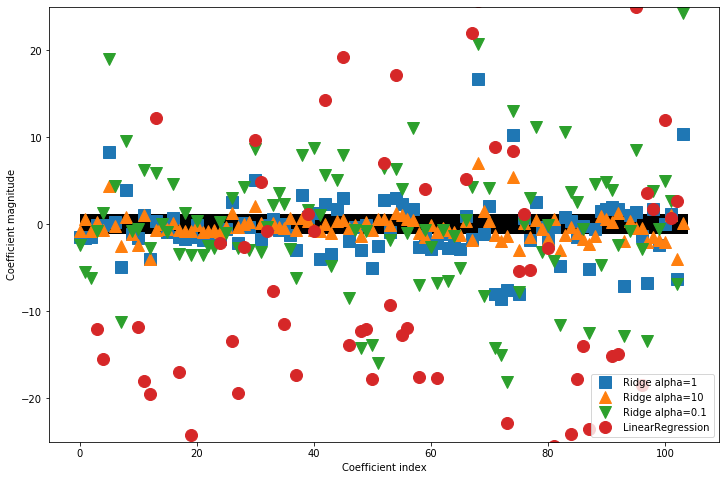alpha越大, 则各个w越接近直线y=0; 对于线性模型, 则点的分布很散.
对比线性回归与岭回归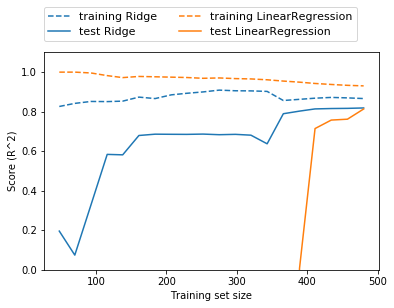线性回归在训练集上的表现始终好于岭回归, 但在训练样本较少的情况下, 线性回归在测试集的表现差, 但随着样本数增多, 线性模型渐渐逼近了岭回归.
在样本数很多的情况下, 正则化是没有必要的.
Lasso
Lasso的正则化是要求某些w恰为0.
import mglearn
import numpy as np
from sklearn.model_selection import train_test_split
from sklearn.linear_model import Lasso

X_train, X_test, y_train, y_test = train_test_split(X, y, random_state=0)

lasso = Lasso(alpha=1.0).fit(X_train, y_train)

print("Training set score: {:.2f}".format(lasso.score(X_train, y_train)))
print("Test set score: {:.2f}".format(lasso.score(X_test, y_test)))
print("Number of features used: {}".format(np.sum(lasso.coef_ != 0)))

alpha默认1, 发生欠拟合, 105个特征只用了4个, 训练集测试集分数只有0.29, 0.21.
更改参数
lasso001 = Lasso(alpha=0.01, max_iter=100000).fit(X_train, y_train)

Training set score: 0.90
Test set score: 0.77
Number of features used: 33
lasso00001 = Lasso(alpha=0.0001, max_iter=100000).fit(X_train, y_train)

Training set score: 0.95
Test set score: 0.64
Number of features used: 94
alpha过小则过拟合.


展开全文• m=Number of training examples h(hypothesis)=output function...Linear regression with one variable. Univariate linear regression.Idea: Choose θ0,θ1\theta_0,\theta_1 so that hθ(x)h_\theta(x)is close to
m=Number of training examples h(hypothesis)=output function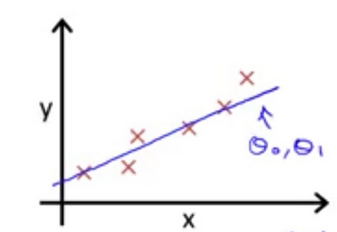Linear regression with one variable. Univariate linear regression.

Idea: Choose θ0,θ1<!--//--><![CDATA[//><!--
\theta_0,\theta_1
//--><!]]> so that hθ(x)<!--//--><![CDATA[//><!--
h_\theta(x)
//--><!]]>is close to y for our training examples(x,y)

Cost Function: squared error function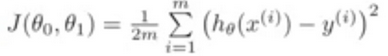minimize J(θ0,θ1<!--//--><![CDATA[//><!--
\theta_0,\theta_1
//--><!]]>)——overall objective for linear regression

outline:

\theta_0,\theta_1
//--><!]]>(initialize them to 0)
(the result depending on where to start on the graph,however J(θ0,θ1<!--//--><![CDATA[//><!--
\theta_0,\theta_1
//--><!]]>) is a convex function)

repeat until convergence{ θj:=θj−αδδθjJ(θ0,θ1)(for j=0 and j=1)<!--//--><![CDATA[//><!--
\theta_j:=\theta_j-\alpha\frac{\delta}{\delta\theta_j}J(\theta_0,\theta_1)\text{(for j=0 and j=1)}
//--><!]]>
} αδδθjJ(θ0,θ1)<!--//--><![CDATA[//><!--
\alpha\frac{\delta}{\delta\theta_j}J(\theta_0,\theta_1)
//--><!]]> is the derivative term α<!--//--><![CDATA[//><!--
\alpha
//--><!]]> is learning rate *simultaneous update temp0:=...<!--//--><![CDATA[//><!--
temp_0:=...
//--><!]]>temp1:=...<!--//--><![CDATA[//><!--
temp_1:=...
//--><!]]>θ0=temp0<!--//--><![CDATA[//><!--
\theta_0=temp_0
//--><!]]>θ1=temp1<!--//--><![CDATA[//><!--
\theta_1=temp_1
//--><!]]>
if you do not do so,maybe it also works well, but we don’t call it GD algorithm.

compress: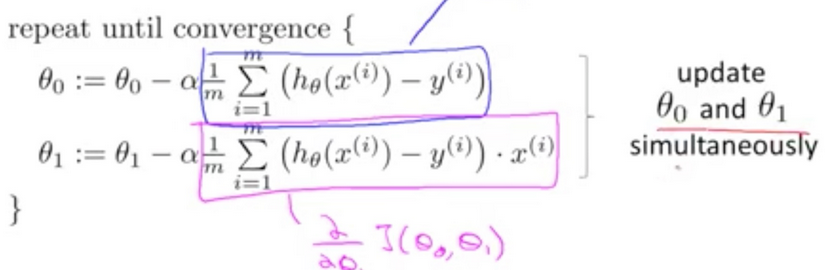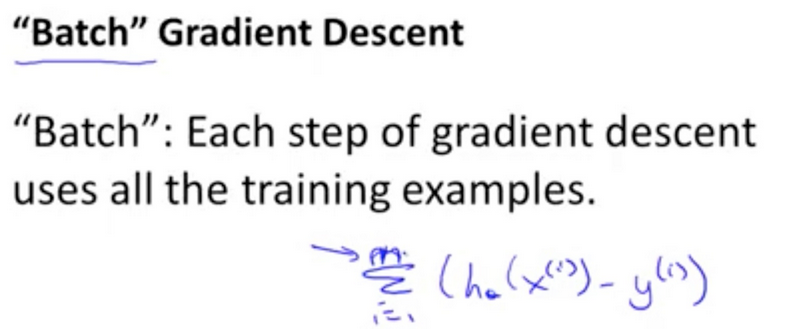if α<!--//--><![CDATA[//><!--
\alpha
//--><!]]> is too small ,gradient descent can be slow. if α<!--//--><![CDATA[//><!--
\alpha
//--><!]]> is too large,gradient decent can overshoot the minimum.It may fail to converge, or even diverge.
GD can converge to a local minimum,even with the learning rate α<!--//--><![CDATA[//><!--
\alpha
//--><!]]> fixed, because as we approach a local minimum, GD will automatically take smaller steps.

Linear Regression with Multiple Features X(i)j<!--//--><![CDATA[//><!--
X_j^{(i)}
//--><!]]> is the value of feature j in ith<!--//--><![CDATA[//><!--
i^{th}
//--><!]]> training example hθ(x)=θ0x0+θ1x1+...θnxn=θTX<!--//--><![CDATA[//><!--
h_\theta(x)=\theta_0x_0+\theta_1x_1+...\theta_nx_n=\theta^TX
//--><!]]>
(both with n+1 elements)
(define x0=1<!--//--><![CDATA[//><!--
x_0=1
//--><!]]>)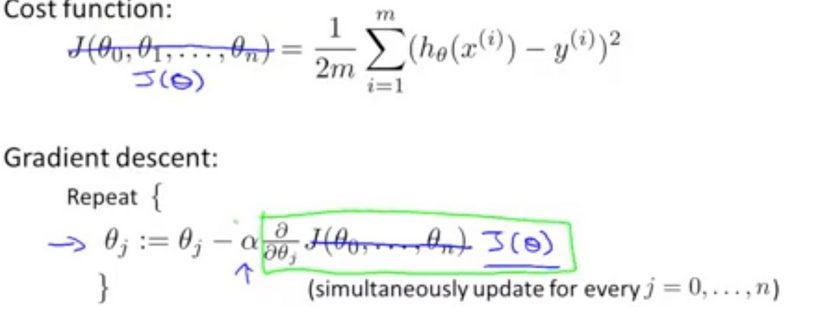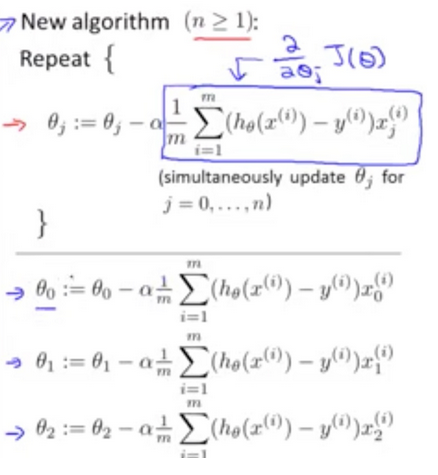feature scaling
if different features takes on similar ranges of values,FD will converge more quickly.
It speeds up gradient descent by making it require fewer iterations to get to a good solution.
maybe
-3 to 3
-13<!--//--><![CDATA[//><!--
\frac{1}{3}
//--><!]]> to 13<!--//--><![CDATA[//><!--
\frac{1}{3}
//--><!]]>
is well
else x1<!--//--><![CDATA[//><!--
x_1
//--><!]]>=...10000<!--//--><![CDATA[//><!--
\frac{...}{10000}
//--><!]]>
get every feature into approximately a −1≤xi≤1<!--//--><![CDATA[//><!--
-1\le x_i \le 1
//--><!]]>
range or standard deviation

mean normalization
makes features have approximately 0

to implement both measures above: x = (value - average_value)/(max_value - min_value)

feature scaling doesn’t have to be too exact

converge judge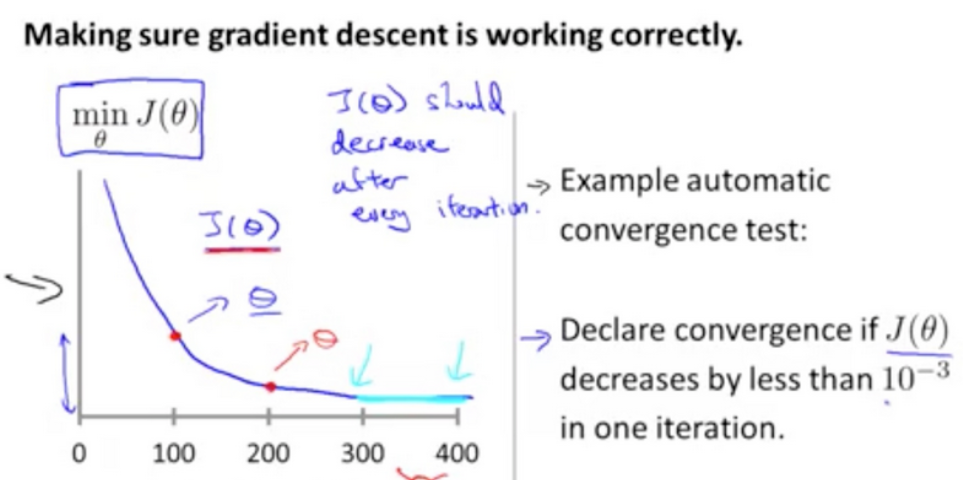There is a example convergence test,but the threshold is not easy to find. So we had better plot the function.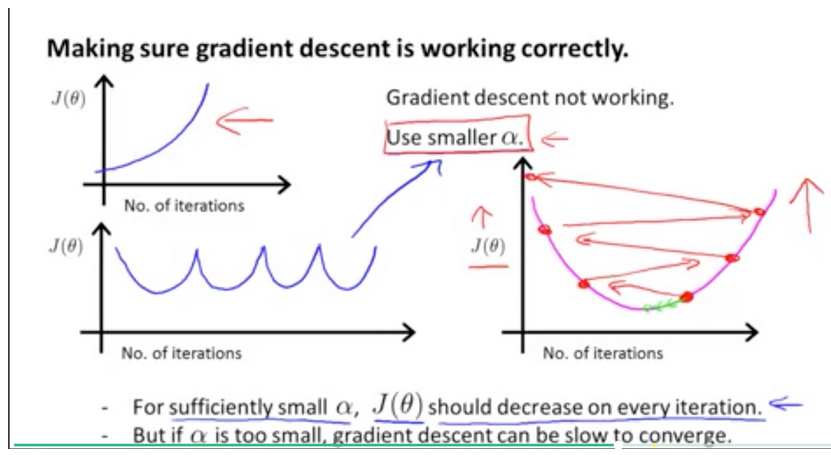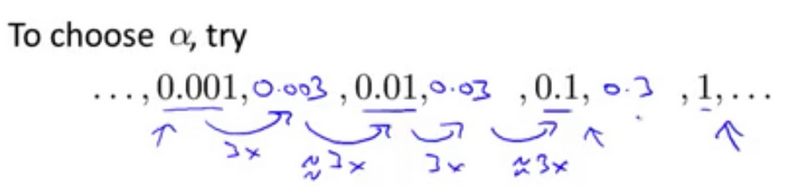展开全文...